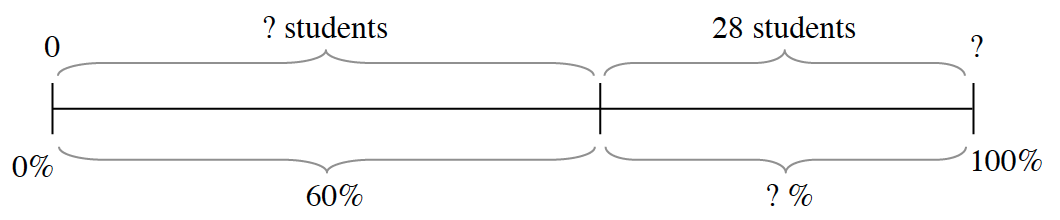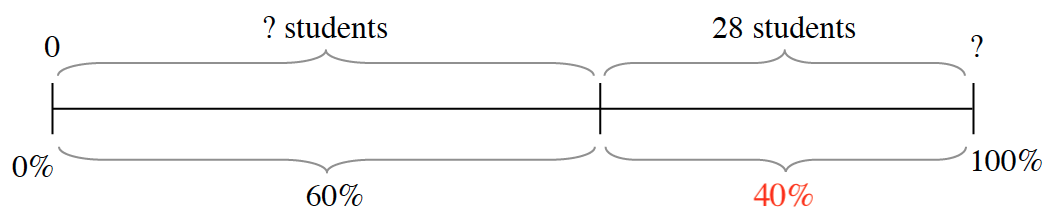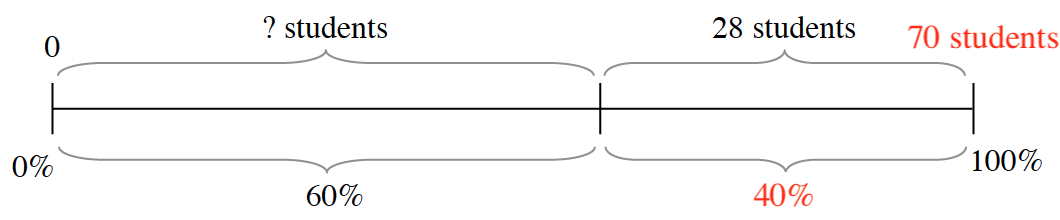### Home > CC2 > Chapter 7 > Lesson 7.1.2 > Problem7-24

7-24.

Copy the diagram below on your paper. Use the given information to fill in all of the missing labels.First, solve for the lower half of the diagram.

Label added to bottom right bracket, 40%. Since the entire diagram is representative of $100\%$ of the students, and the first portion represents $60\%$, the second portion must represent $40\%$$28$ students is $40\%$ of how many total students?

$\frac{40}{100}=\frac{28}{x}$Label added to top right end, 70 students.

To find the remaining number of students for the first portion, subtract $28$ from the total number of students.